# ADC on STM8S using Cosmic C Compiler – Reading Multiple ADC Values and Displaying on LCD

Published  October 15, 2020   3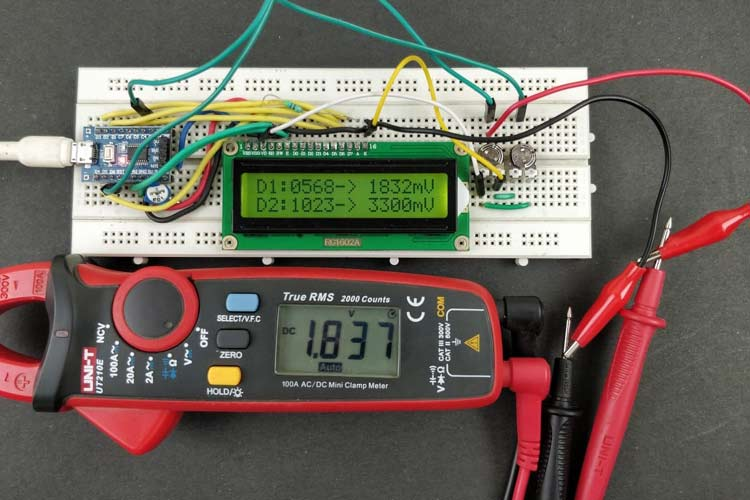If you are a regular reader who is following our STM8S Microcontroller Tutorials, you would know that in our last tutorial, we learned how to interface a 16x2 LCD with STM8s. Now, proceeding with it on this tutorial, we will learn how to use the ADC feature on our STM8S103F3P6 Microcontroller. An ADC is a very useful peripheral on a microcontroller that is often used by embedded programmers to measure units that are in constant change like the varying voltage, current, temperature, humidity, etc.

As we know “We live in an analog world with digital devices”, meaning everything around us like wind speed, light intensity, temperature, and everything we deal with like speed, velocity, pressure, etc. are analog in nature. But our microcontrollers and microprocessors are digital devices and they will not be able to measure these parameters without an important peripheral called Analog to Digital Converters (ADC). So in this article, let's learn how to use ADC on STM8S Microcontroller with COMIC C compiler.

### Materials Required

In this article, we will be reading two analog voltage values from two potentiometers and display its ADC value on a 16x2 LCD display. To do this, we will need the following components.

• STM8S103F3P6 Development board
• 16x2 LCD
• Potentiometers
• Connecting wires
• 1k resistor

There are many types of ADC and each microcontroller has its own specifications. On the STM8S103F3P6, we have an ADC with 5 Channel and 10-bit resolution; with a 10-bit resolution, we will be able to measure the digital value from 0 to 1024 and a 5 channel ADC indicates that we have 5 pins on the Microcontroller which can support ADC, these 5 pins are highlighted in the picture below.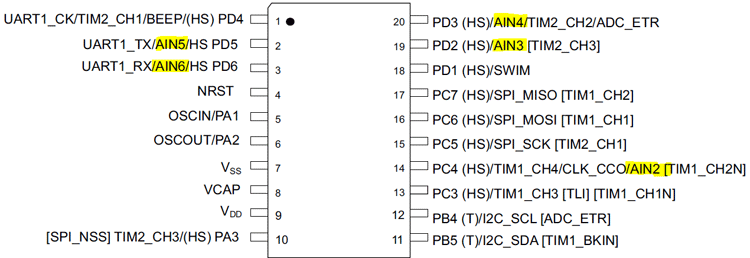As you can see, all these five pins (AIN2, AIN3, AIN4, AIN5, and AIN6) are multiplexed with other peripherals, meaning apart from just acting as an ADC pin, these pins can also be used for performing other communications like for example, the pin 2 and 3 (AIN5 and AIN 6) can not only be used for ADC but can also be used for serial communication and GPIO functions. Do note that it will not be possible to use the same pin for all three purposes, so if we are using these two pins for ADC, then we will not be able to perform serial communication. Other important ADC characteristics for STM8S103P36 can be found in the below table taken from the datasheet.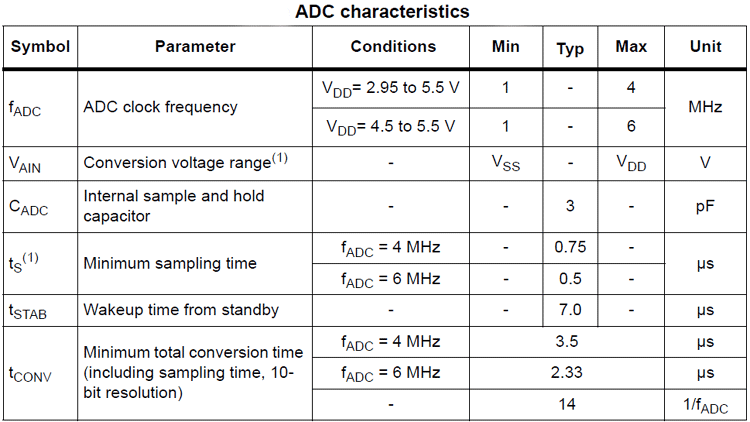In the above table, Vdd represents operating voltage and Vss represents the ground. So in our case on our development board, we have the microcontroller operating on 3.3V, you can check the development board circuit diagram from the getting started with the STM8S tutorial. With 3.3V as the operating voltage, our ADC clock frequency can be set between 1 to 4MHz and our conversion voltage range is between 0V to 3.3V. This means that our 10-bit ADC will read 0 when 0V(Vss) is provided and will read a maximum of 1024 when 3.3V(Vdd) is provided. We can easily change this 0-5V by changing the operating voltage of the MCU if required.

### Circuit Diagram to Read ADC Values on STM8S  and Display on LCD

The complete circuit diagram used in this project is given below, it is very similar to the STM8S LCD tutorial that we discussed previously.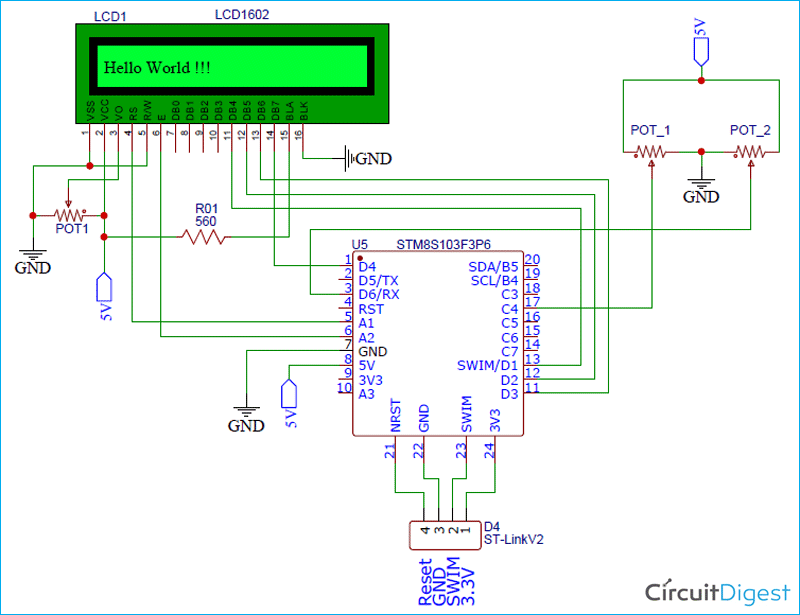As you can see, the only additional components apart from the LCD are two potentiometers POT_1 and POT_2. These pots are connected to the ports PC4 and PD6, which are the ANI2 and ANI6 pins as discussed on the pinout image earlier.

The potentiometers are connected in such a way that when we vary it, we will get 0-5 V on our analog pins. We will be programming our controller to read this analog voltage in digital value (0 to 1024) and display it on the LCD screen. Then we will also calculate the equivalent voltage value and display it on the LCD, do remember that our controller is powered by 3.3V, so even if we provide 5V to the ADC pin, it will be able to read only from 0V to 3.3V.

Once the connections are done, my hardware looks like this as shown below. You can see the two potentiometers on the right and the ST-link programmer on the left.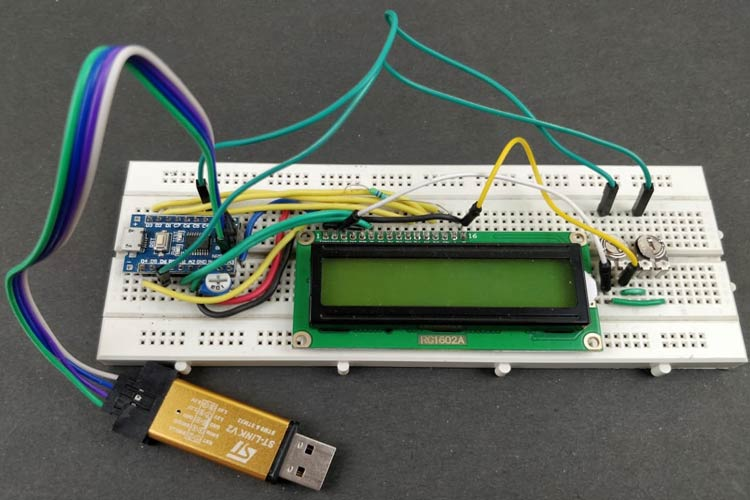To program for ADC functionalities on STM8S, we will be using the Cosmic C compiler along with the SPL libraries. But for making the processes easier, I made another header file that can be found on GitHub with the link below.

If you know what you are doing, you can create a header file using the above code and add it to the “include files” directory on your project page. Else follow the getting started with the STM8S tutorial to know how to set-up your programming environment and compiler. Once your setup is ready, your IDE should have the following header files, at least the ones encircled in red.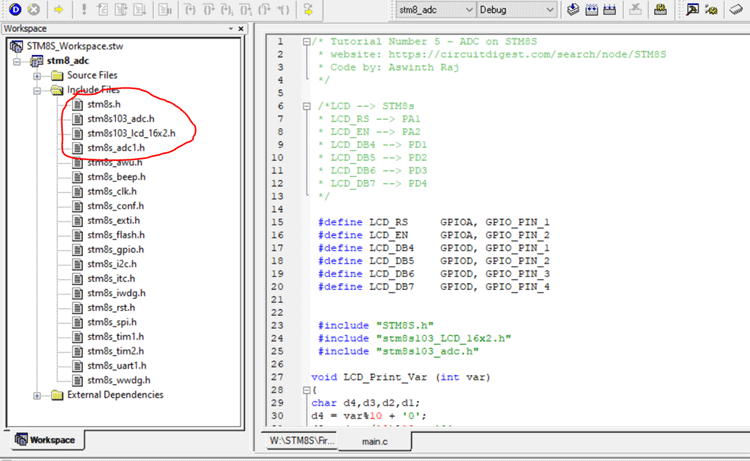The above header file consists of a function called ADC_Read(). This function can be called in your main program to get the ADC value at any pin. For example, ADC_Read(AN2) will return the ADC value on pin AN2 as result. The function is shown below.

```unsigned int ADC_Read(ADC_CHANNEL_TypeDef ADC_Channel_Number)
{
unsigned int result = 0;
DISABLE,
DISABLE);

As you see, we can pass eight parameters to this function and this defines how the ADC is configured. In our library code above, we have set the conversion mode to continuous and then get channel number passed a parameter. And then we have to set the CPU frequency of our controller, by default (if you have not connected an external crystal), your STM8S will work with a 16Mhz internal oscillator. So we have mentioned “ADC1_PRESSEL_FCPU_D18” as the pre-scaler value. Inside this function, we are using other methods defined by the SPL stm8s_adc1.h header file. We start by De-initializing the ADC pins and then ADC1_Init () to initialize the ADC peripheral. The definition of this function from the SPL user manual is shown below.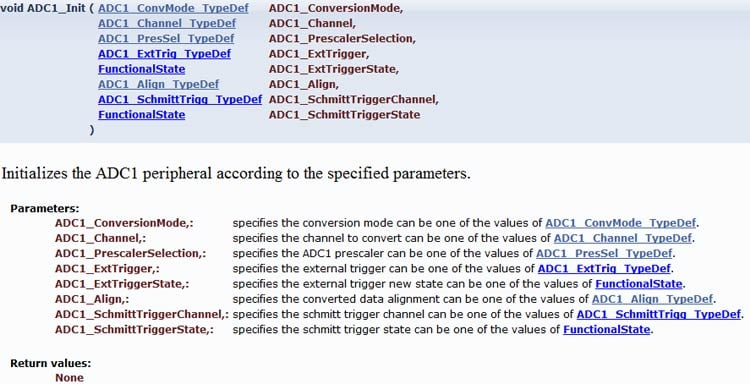Next, we set the external trigger using a timer and disable external trigger since we won’t be using it here. And then we have the alignment set to the right and the last two parameters are used to set Schmitt trigger, but we will be disabling it for this tutorial. So, to put it short, we will our ADC working in continuous conversion mode on the required ADC pin with external trigger and Schmitt trigger disabled. You can check the datasheet if you need more information on how to use the external trigger or Schmitt trigger option, we won’t be discussing that in this tutorial.

### STM8S Program to Read Analog Voltage and Display on LCD

The complete code used in the main.c file can be found at the bottom of this page. After adding the required header files and source files, you should be able to directly compile the main file. The explanation of the code in the main file is as follows. I will not be explaining the STM8S LCD program since we have already discussed that in the previous tutorial.

The purpose of the code will be to read ADC values from two pins and convert it to a voltage value. We will also display both the ADC value and Voltage value on the LCD. So, I have used a function called LCD_Print Var which takes in a variable in integer format and converts it to a character so as to display it on the LCD. We have used the simple modulus (%) and divide (/) operators to get each digit from the variable and put in variables like d1,d2,d3, and d4 as shown below. Then we can use the LCD_Print_Char function to display these characters on the LCD.

```void LCD_Print_Var (int var)
{
char d4,d3,d2,d1;
d4 = var%10 + '0';
d3 = (var/10)%10 + '0';
d2 = (var/100)%10 + '0';
d1 = (var/1000) + '0';
Lcd_Print_Char(d1);
Lcd_Print_Char(d2);
Lcd_Print_Char(d3);
Lcd_Print_Char(d4);
}```

Next under the main function, we have four variables declared. Two of them are used to save the ADC value (0 to 1024) and the other two are used to get the actual voltage value.

```unsigned int ADC_value_1 = 0;

Next, we have to prepare the GPIO pins and clock configuration to read analog voltage. Here we will be reading the analog voltage from pins AIN2 and AIN6 which are pins PC4 and PD6 respectively. We have to define these pin in a floating state as shown below. We will also be enabling the clock peripheral for ADC.

```CLK_PeripheralClockConfig(CLK_PERIPHERAL_ADC, ENABLE); //Enable Peripheral Clock for ADC
GPIO_Init (GPIOC, GPIO_PIN_4, GPIO_MODE_IN_FL_IT);
GPIO_Init (GPIOC, GPIO_PIN_4, GPIO_MODE_IN_FL_IT);```

Now that the pins are ready, we have to get into the infinite while loop to read the analog voltage. Since we have our header file, we can easily read the analog voltage from pins AIN2 and AIN 6 using the below lines.

```ADC_value_1 = ADC_Read (AIN2);

The next step is to convert this ADC reading (0 to 1023) to an analog voltage. This way, we can display the exact voltage value given to pin AIN2 and AIN6. The formulae to calculate Analog Voltage can be given by-

`Analog Voltage  = ADC Reading * (3300/1023)`

In our case on STM8S103F3 controllers, we have an ADC with 10-bit resolution, so we have used 1023 (2^10). Also on our development powers the controller with 3.3V which is 3300, so we divided 3300 by 1023 in the above formulae. Approximately 3300/1023 will give us 3.226, so on our program, we have the following lines to measure the actual ADC voltage using the ADC voltage.

```ADC_voltage_1 = ADC_value_1 * (3.226); //(3300/1023 =~ 3.226)convert ADC value 1 to 0 to 3300mV

The remaining part of the code is only used to display these four values on the LCD screen. We also have a delay of 500ms so that the LCD gets updated for every 500mS. You can reduce this further if you need faster updates.

### Reading Analog Voltage from Two Potentiometer using STM8S

Compile the code and upload it to your development board. If you get any compiling error, make sure you have added all the header files and source files as discussed earlier. Once the code is uploaded, you should see a small welcome message saying “ADC on STM8S” and then you should see the below screen.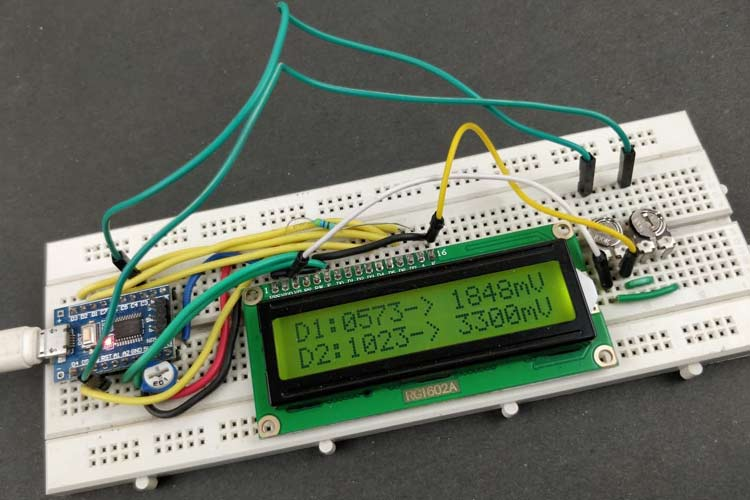The value D1 and D2 indicate the ADC value from pin Ain2 and AIN6 respectively. On the right side, we also have the equivalent voltage values displayed. This value should be equal to the voltage appearing on pin AIN2 and AIN6 respectively. We can check for the same using a multimeter, we can also vary the potentiometers to check if the voltage value also changes accordingly.Complete working can also be found in the video below. Hope you enjoyed the tutorial and learned something useful, if you have any questions, leave them in the comment section below. You can also use our forums to start a discussion or post other technical questions.

Code
```/* Tutorial Number 5 - ADC on STM8S
* website: https://circuitdigest.com/search/node/STM8S
* Code by: Aswinth Raj
*/
/*LCD --> STM8s
* LCD_RS --> PA1
* LCD_EN --> PA2
* LCD_DB4 --> PD1
* LCD_DB5 --> PD2
* LCD_DB6 --> PD3
* LCD_DB7 --> PD4
*/
#define LCD_RS     GPIOA, GPIO_PIN_1
#define LCD_EN     GPIOA, GPIO_PIN_2
#define LCD_DB4    GPIOD, GPIO_PIN_1
#define LCD_DB5    GPIOD, GPIO_PIN_2
#define LCD_DB6    GPIOD, GPIO_PIN_3
#define LCD_DB7    GPIOD, GPIO_PIN_4
#include "STM8S.h"
#include "stm8s103_LCD_16x2.h"
void LCD_Print_Var (int var)
{
char d4,d3,d2,d1;
d4 = var%10 + '0';
d3 = (var/10)%10 + '0';
d2 = (var/100)%10 + '0';
d1 = (var/1000) + '0';
Lcd_Print_Char(d1);
Lcd_Print_Char(d2);
Lcd_Print_Char(d3);
Lcd_Print_Char(d4);
}
void main()
{
//Variable declarations
Lcd_Begin();
Lcd_Clear();
Lcd_Set_Cursor(1,1);
GPIO_Init (GPIOC, GPIO_PIN_4, GPIO_MODE_IN_FL_IT);
delay_ms(5000);
Lcd_Clear();
Lcd_Set_Cursor(1,1);
Lcd_Print_String("D1:    ->     mV");
Lcd_Set_Cursor(2,1);
Lcd_Print_String("D2:    ->     mV");
while (1)
{
Lcd_Set_Cursor(1,4);
Lcd_Set_Cursor(1,11);
Lcd_Set_Cursor(2,4);
Lcd_Set_Cursor(2,11);
delay_ms(500);
}
}```
Video

Tags